Next: Vector Calculus Up: Vectors Previous: The Scalar Product

## The Vector Product

We have discovered how to construct a scalar from the components of two general vectors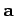and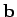. Can we also construct a vector which is not just a linear combination ofand? Consider the following definition: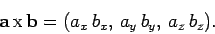(27)

Is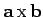a proper vector? Suppose that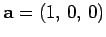and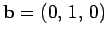. Clearly,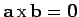. However, if we rotate the basis through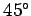about the-axis then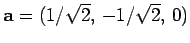,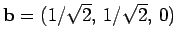, and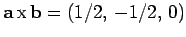. Thus,does not transform like a vector, because its magnitude depends on the choice of axes. So, above definition is a bad one.

Consider, now, the cross product or vector product,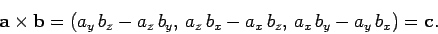(28)

Does this rather unlikely combination transform like a vector? Let us try rotating the basis throughdegrees about the-axis using Eqs. (10)-(12). In the new basis,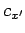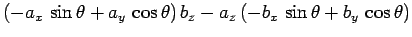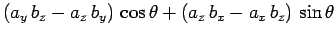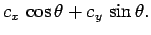(29)

Thus, the-component of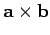transforms correctly. It can easily be shown that the other components transform correctly as well, and that all components also transform correctly under rotation about the- and-axes. Thus,is a proper vector. Incidentally,is the only simple combination of the components of two vectors which transforms like a vector (which is non-coplanar withand). The cross product is anticommutative,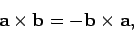(30)

distributive,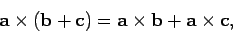(31)

but is not associative: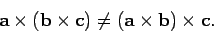(32)

Note thatcan be written in the convenient, and easy to remember, determinant form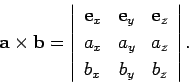(33)

The cross product transforms like a vector, which means that it must have a well-defined direction and magnitude. We can show thatis perpendicular to bothand. Consider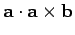. If this is zero then the cross product must be perpendicular to. Now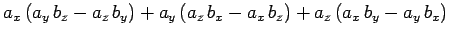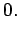(34)

Therefore,is perpendicular to. Likewise, it can be demonstrated thatis perpendicular to. The vectors,, andform a right-handed set, like the unit vectors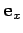,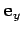, and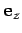. In fact,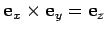. This defines a unique direction for, which is obtained from a right-hand rule--see Fig. 6.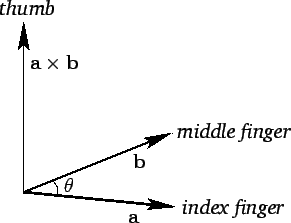Let us now evaluate the magnitude of. We have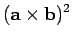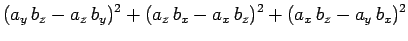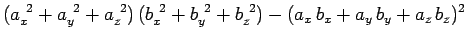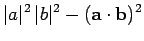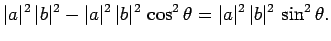(35)

Thus,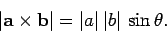(36)

Clearly,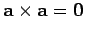for any vector, sinceis always zero in this case. Also, if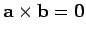then either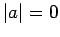,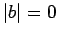, oris parallel (or antiparallel) to.

Suppose that a force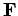is applied at position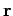--see Fig. 7. The moment, or torque, about the origin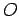is the product of the magnitude of the force and the length of the lever arm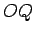. Thus, the magnitude of the moment is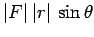. The direction of the moment is conventionally the direction of the axis throughabout which the force tries to rotate objects, in the sense determined by a right-hand grip rule. It follows that the vector moment is given by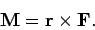(37)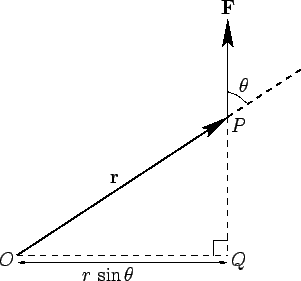Next: Vector Calculus Up: Vectors Previous: The Scalar Product
Richard Fitzpatrick 2007-07-14